# Reasoning Quiz For RRB NTPC : 2nd January

Q1. Which of the following interchanges in signs will make the given equation correct?
17- 15 x 5 = 250
(a) = and x
(b) + and –
(c) = and –
(d) x and –

Q2. There are five boys Prem, Raju, Sundar,Hari and Ompal. Raju has more share of land than Sundar but lesser than Prem.Ompal has the least share and Hari has lesser share of land than Sundar. Who owns the highest share of land?
(a) Sundar
(b) Raju
(c) Hari
(d) Prem

Q3. Arrange the given words in the sequence in which they occur in the dictionary.
i. Permanence
ii. Permanent
iii. Permafrost
iv. Permeability
(a) ii, iii, iv, i
(b) iii, ii, i, iv
(c) iv, ii, i, iii
(d) iii, i, ii, iv

Q4. If a mirror is placed on the line MN, then which of the answer figures is the right image of the given figure?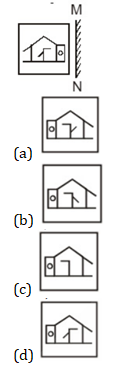Q5. A man is facing west and he turns anti-clockwise by 90 degrees and moves 10m straight, then he again turns 90 degrees anti-clockwise. Which direction is the man facing now?
(a) North
(b) West
(c) South
(d) East

Q6. Identify the diagram that best represents the relationship among the given classes.
Football, Volleyball, Outdoor Games, Carrom.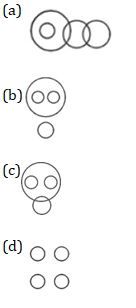Q7. Aarush’s birthday is on Thursday 27th April. On what day of the week will be Mitul’s Birthday in the same year if Mitul was born on 28th September?
(a) Monday
(b) Thursday
(c) Wednesday
(d) Tuesday

Q8. The weights of 4 boxes are 20, 30, 40 and 60 kilograms. Which of the following cannot be the total weight, in kilograms, of any combination of these boxes and in a combination a box can be used only once?
(a) 150
(b) 110
(c) 130
(d) 140

Q9. If 9#3=6; 15#3=9; 60#4=32; then what is the value of 27#3=?
(a) 24
(b) 15
(c) 13
(d) 33

Q10. In the question two statements are given, followed by two conclusions, I and II. You have to consider the statements to be true even if it seems to be at variance from commonly known facts. You have to decide which of the given conclusions, if any, follows from the given statements.
Statement 1 : No squares are rectangles.
Statement 2 : All rectangles are triangles.
Conclusion I : Some triangles are squares.
Conclusion II :Some triangles are rectangles.
(a) Only conclusion I follows
(b) Only conclusion II follows
(c) Both I and II follow
(c) Neither I nor II follows

Solutions: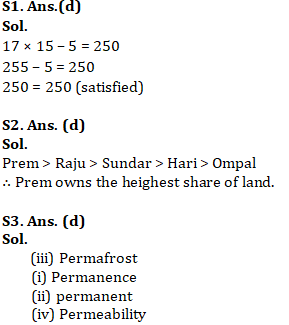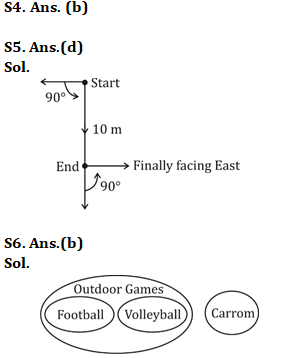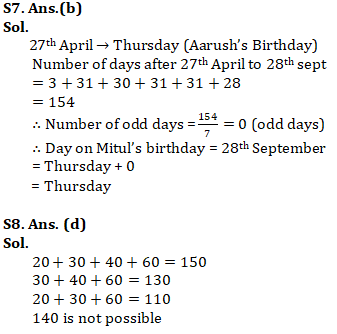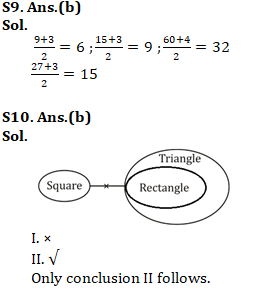Important Links for RRB NTPC Recruitment 2019